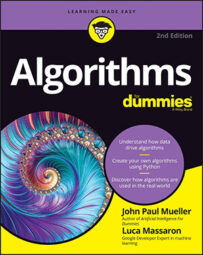##### Algorithms For DummiesIf you're like most people, you often find yourself scratching your head when it comes to math structures because no one seems to know how to use the terms correctly. It's as though people are purposely trying to make things hard! After all, what is an equation and why is it different from an algorithm? Well, fear no more: The following table provides the definitive guide to math structures that you might encounter but have been afraid to ask about.
 Structure Description Equation Numbers and symbols that, when taken as a whole, equate to a specific value. An equation always contains an equals sign so that you know that the numbers and symbols represent the specific value on the other side of the equals sign. Equations generally contain variable information presented as a symbol, but they aren't required to use variables. Formula A combination of numbers and symbols used to express information or ideas. A formula normally presents mathematical or logical concepts, such as to define the Greatest Common Divisor (GCD) of two integers (the video at Khan Academy tells how this works). Generally, a formula shows the relationship between two or more variables. Most people see a formula as a special kind of equation. Algorithm A sequence of steps used to solve a problem. The sequence presents a unique method of addressing an issue by providing a particular solution. An algorithm need not represent mathematical or logical concepts, even though the presentations in this book often do fall into that category because people most commonly use algorithms in this manner. Some special formulas are also algorithms, such as the quadratic formula. For a process to represent an algorithm, it must be the following:Finite: The algorithm must eventually solve the problem.Well-defined: The series of steps must be precise and present steps that are understandable, especially by computers, which must be able to to create a usable algorithm.Effective: An algorithm must solve all cases of the problem for which someone defined it. An algorithm should always solve the problem it has to solve. Even though you should anticipate some failures, the incidence of failure is rare and occurs only in situations that are acceptable for the intended algorithm use.